# Continuous Functions Theorems

Lesson Transcript
Instructor
Usha Bhakuni

Usha has taught high school level Math and has master's degree in Finance

Expert Contributor
Kathryn Boddie

Kathryn has taught high school or university mathematics for over 10 years. She has a Ph.D. in Applied Mathematics from the University of Wisconsin-Milwaukee, an M.S. in Mathematics from Florida State University, and a B.S. in Mathematics from the University of Wisconsin-Madison.

Continuous functions are functions that have their conditions satisfied between multiple points, appearing as an uninterrupted line when graphed. See examples of how this is represented in the intermediate value theorem and the extreme value theorem. Updated: 11/04/2021

## Continuous functions

A continuous function in mathematics is defined as a function that is defined at each point in its domain. Basically, a function whose graph is an unbroken curve in its domain is a continuous function.

In mathematical terms, a function f(x) is considered to be continuous at a point c if and only if it satisfies the following conditions: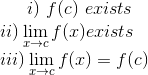The functions that are continuous at every point in their domain are continuous functions.

Let's see an example: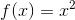The domain of this function is all real numbers [- ∞, + ∞]. This function satisfies all three conditions, so it is a continuous function, as can be seen in its graph.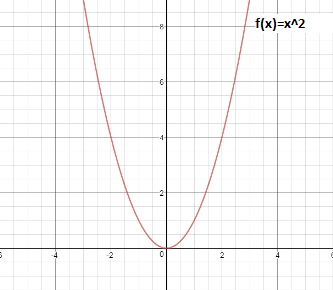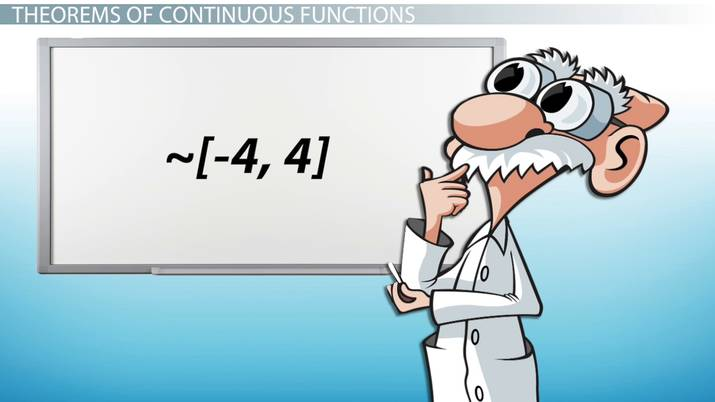An error occurred trying to load this video.

Try refreshing the page, or contact customer support.

Coming up next: Intermediate Value Theorem: Definition

### You're on a roll. Keep up the good work!

Replay
Your next lesson will play in 10 seconds
• 0:03 Continuous Functions
• 0:57 Theorems of Continuous…
• 2:33 Lesson Summary
Save Save

Want to watch this again later?

Timeline
Autoplay
Autoplay
Speed Speed

## Theorems of Continuous Functions

We will now look at two important theorems pertaining to continuous functions: the intermediate value theorem and the extreme value theorem.

### 1. Intermediate Value Theorem

For the intermediate value theorem, let's assume a function f(x) that is continuous between [a,b]. Suppose there is a number p between f(a) and f(b). This theorem states that there must be at least one value q between a and b, such that f(q) = p.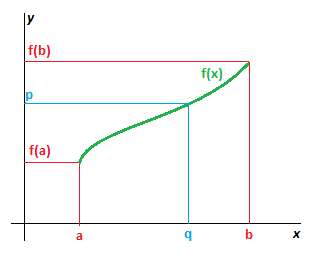Taking the example of the previous function,Between the range [0,4], there exists a value f(q) = 4 for q = 2, which can be illustrated like this: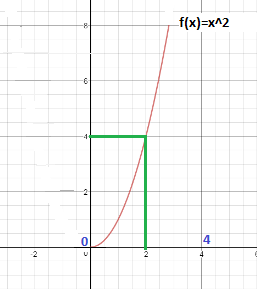There could be functions that have more than one point between a and b with the same value. Let's consider an interval of [-4,4] for our previous example. In this case, we get the value f(q) = 4 at two points: q = -2 and q = +2, as we can see in this graph.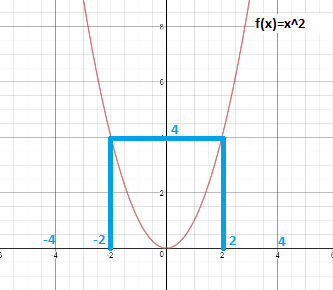To unlock this lesson you must be a Study.com Member.

## Continuous Functions Theorems - Examples and Counterexamples

In the following problems, students will apply their knowledge of the continuous function theorems to solve for the values satisfying the theorems and to construct counterexamples.

## Problems

1) Verify that f(x) = 3x^2 - 2x is continuous, and find a value (q) satisfying the Intermediate Value Theorem for f(q) = 5, using the fact that f(0) = 0 and f(2) = 8.

2) Graph the function f(x) = 3x + 2 on the interval [0,3] and verify that the Extreme Value Theorem is satisfied.

3) Show that the function f(x) = x^3 + x - 2 has a root in the interval [0,2].

4) Create an example of a discontinuous function which doesn't satisfy the Intermediate Value Theorem.

## Solutions

1) The function is continuous, as seen on the graph: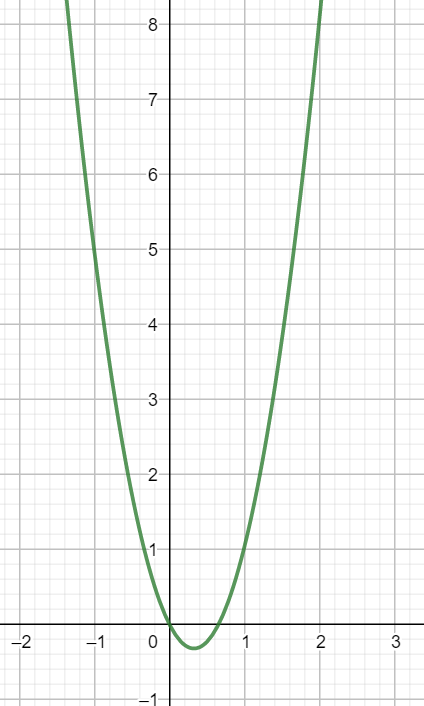We know a value for q exists by the Intermediate Value Theorem. To find q, we need to solve the equation 3q^2 - 2q = 5 , or equivalently, 3q^2 - 2q - 5 = 0. Factoring gives us (q + 1) (3q - 5) = 0 and so q = -1 or q = 5/3. The value we want is q = 5/3 since 0 < 5/3 < 2.

2) The graph of the function on the interval is below: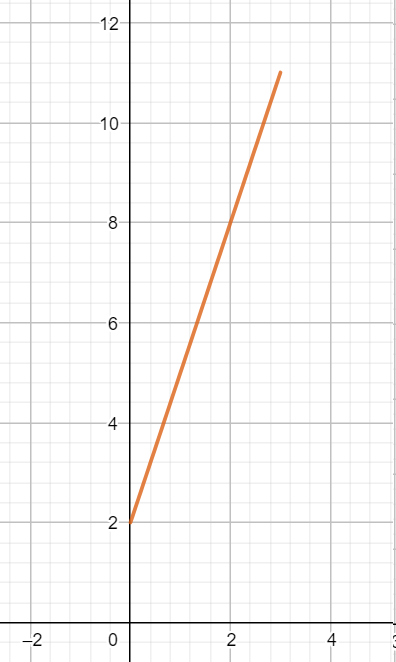The Extreme Value Theorem is satisfied - we have a continuous function on a closed interval and the absolute maximum is 11 at x = 3 and the absolute minimum is 2 at x = 0.

3) Using the end points, we see that f(0) = 0^3 + 0 - 2 = -2 and f(2) = 2^3 + 2 - 2 = 8. The Intermediate Value Theorem says that for any f(0)< p < f(2) there exists 0 < q < 2, such that f(q) = p. Since p = 0 is a value between f(0) and f(2), there exists a 'q' with f(q) = 0; that is, there is a root.

4) One possible example is the piece-wise function f(x) = 2 when x is in the interval [0,1] and f(x) = 0 when x is interval (1,2]. The function is graphed below and the Intermediate Value Theorem is not satisfied. For example, a value between f(0) and f(2) is 1, but there is no x value for which f(q) = 1.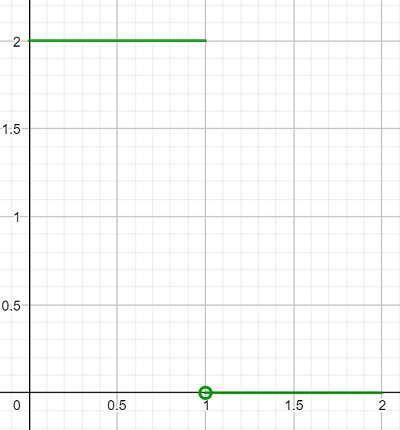### Register to view this lesson

Are you a student or a teacher?